Subject:
Numbers and Operations
Material Type:
Lesson Plan
Level:
Middle School
7
Provider:
Pearson
Tags:
Language:
English
Media Formats:
Text/HTML

# Calculating Ranges Of Strouhal Numbers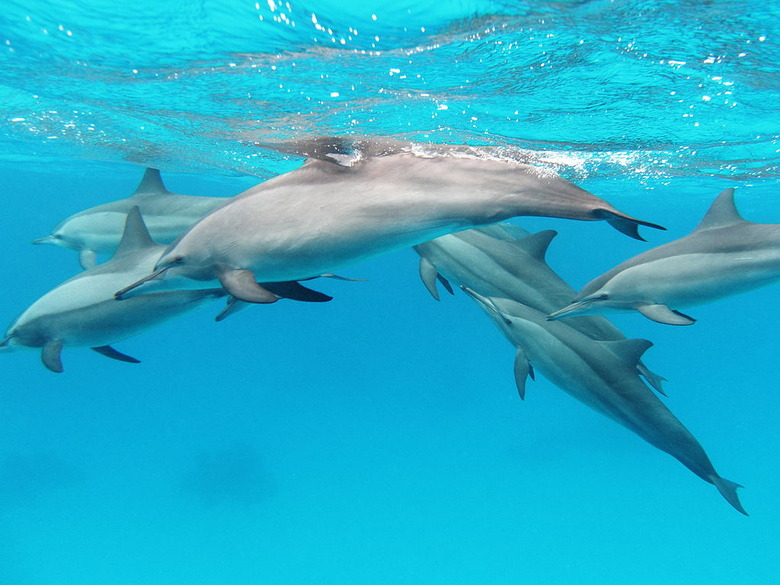## Overview

Students create a bar graph showing the Strouhal numbers for a variety of birds and bats and use their graph and other data to compare the Strouhal numbers of the different animals to analyze variation and to make predictions.

# Key Concepts

Students are expected to use the mathematical skills they have acquired in previous lessons or in previous math courses. The lessons in this unit focus on developing and refining problem-solving skills. Students will:

• Try a variety of strategies to approaching different types of problems.
• Devise a problem-solving plan and implement their plan systematically.
• Become aware that problems can be solved in more than one way.
• See the value of approaching problems in a systematic manner.
• Communicate their approaches with precision and articulate why their strategies and solutions are reasonable.
• Make connections between previous learning and real-world problems.
• Create efficacy and confidence in solving challenging problems in a real-world setting.

# Goals and Learning Objectives

• Analyze the relationship among the variables in an equation.
• Write formulas to show how variables relate.
• Calculate ranges of Strouhal numbers and use these ranges to make predictions.
• Communicate findings using multiple representations including tables, charts, graphs, and equations.
• Create bar graphs.

# Lesson Guide

Have students predict the range where they think all birds have Strouhal numbers that are close to each other. Allow the students to discuss their prediction with a partner.

ELL: ELLs may need clarification as to what the question asks them to do. They are not asked to solve a problem, only to make a prediction. Eventually, they will need to review the problem solving process. Make sure that the problems solving process is written on an anchor chart and placed in a prominent location.

# How Do Birds Compare?

Predict the range where you think all birds have Strouhal numbers that are close to each other.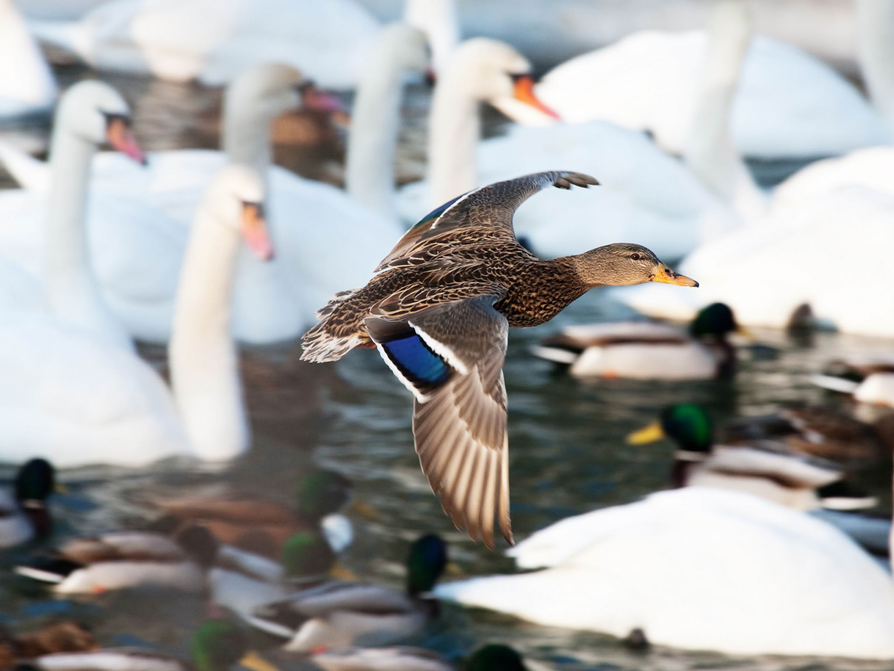# Lesson Guide

Remind students of Problem-Solving Steps 1–4.

1. Understand the problem situation.
• What is the problem asking you to find out?
• What information is provided?
• What are the quantities that vary?
• How are the variables related?
2. Represent the situation.
• Write a formula to show how the variables of the Strouhal number relate to each other.
4. Check that the mathematical answer makes sense.

# Problem-Solving Process

As you solve today's problems, use the problem-solving process.

1. Understand the problem situation.
2. Represent the situation.
4. Check that the mathematical answer makes sense.

# Lesson Guide

Discuss the Math Mission. Students will create a graph showing the Strouhal numbers for different birds and bats and then compare the data to the Strouhal number for a dolphin.

SWD: Students have learned and retained information from prior learning experiences, but do not realize when to use that information. Teachers need to remind students of what they know, but also when to apply that knowledge. This strategy is sometimes referred to as “priming” background information. Priming background knowledge can be done in simple ways, such as merely stating “Remember when you learned how to graph...” or “See how this concept applies in this situation, too?”

## Opening

Create a graph showing the Strouhal numbers for different birds and bats and then compare the data to the Strouhal number for a dolphin.

# Lesson Guide

Have students work independently to start. During independent time, observe students working, but refrain from asking guiding questions or giving students hints. Give students the opportunity to organize their problem-solving plans and the information given in the problem independently before asking others for assistance.

After independent work time, allow students to work with a partner to complete the problems. Have partners share their problem-solving plans. Partners should agree on a plan and work together to find the solutions. During this time, circulate around the classroom asking guiding questions and noting strategies you want students to share during the class discussion.

SWD: Students with disabilities may require review and reinforcement of mathematical tools and means of representing data. Reinforce how to interpret and utilize graphing tools.

# Mathematical Practices

Mathematical Practice 1: Make sense of problems and persevere in solving them.

Students must make sense of the Strouhal numbers for each of the animals and what the range of numbers means for other birds and bats. Students must preserve in solving this problem to make predictions about other types of animals.

Mathematical Practice 2: Reason abstractly and quantitatively.

Students reason abstractly and quantitatively with the Strouhal numbers for each type of bird and bat. Students use their quantitative reasoning skills to calculate the range in the Strouhal numbers and use this data to make predictions.

Mathematical Practice 3: Construct viable arguments and critique the reasoning of others.

Students construct a viable argument for the reasonableness of their solution and they have an opportunity to critique the work of others during the class discussion.

Mathematical Practice 4: Model with mathematics.

Students model the mathematics presented in the problem graphically.

Mathematical Practice 5: Use appropriate tools strategically.

Students use appropriate tools and problem-solving strategies to find the solution. Students may use a calculator as a possible tool for finding their solution.

Mathematical Practice 6: Attend to precision.

Students attend to precision while creating their graphs as well as when calculating the range in Strouhal numbers. Students also use precision when calculating the Strouhal number of a dolphin.

Mathematical Practice 7: Look for and make use of structure.

Students make use of the problem-solving structure while solving this problem.

# Interventions

Student has difficulty getting started.

• What information is provided in the problem? What are you trying to find?
• How can you organize the information presented in the problem?
• What problem-solving strategies can you use to help you find a solution?

Student has a solution, but it is incorrect.

• Does the information in your graph match the data in the table?
• How do you calculate the range for the data?

Student has a solution but is having difficulty articulating his or her thinking.

• How can you state the answer as a complete sentence?
• How would you describe your strategy to somebody who is struggling?

Student has a correct solution and is waiting on others to finish.

• Do you think the Strouhal numbers for all swimming animals would fall within the same range as birds and bats? Why or why not?

• The maximum Strouhal Number in the data is 0.4. The minimum Stouhal Number in the data is 0.2. Therefore, the range of the Strouhal numbers for the birds and bats is 0.2.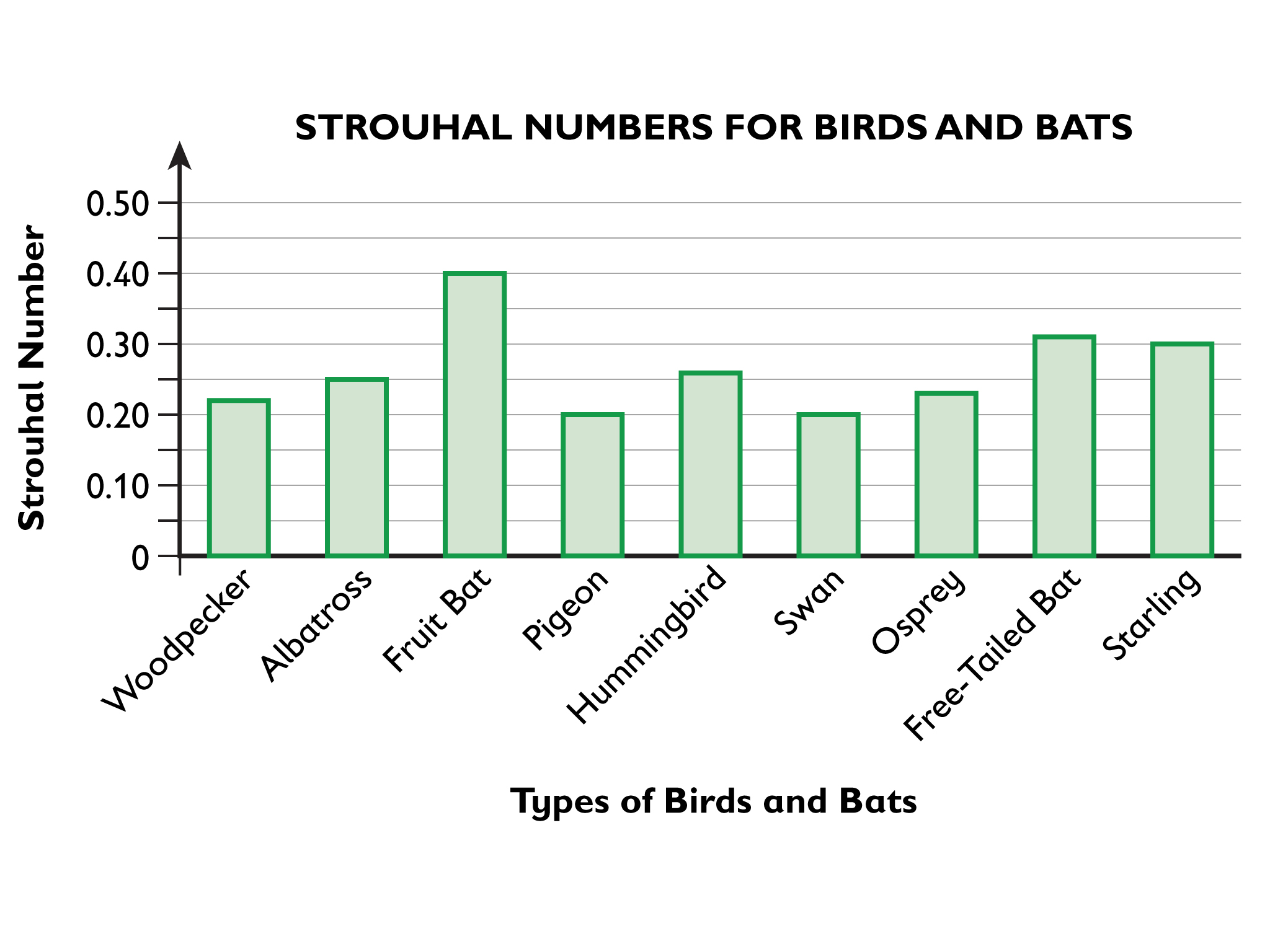# Graph and Compare Strouhal Numbers

• Create a graph that shows the given Strouhal numbers for the following birds and bats.
• Woodpecker: 0.22
• Albatross: 0.25
• Fruit bat: 0.40
• Pigeon: 0.20
• Hummingbird: 0.259
• Swan: 0.20
• Osprey: 0.23
• Free-tailed bat: 0.31
• Starling: 0.30
• What is the range of Strouhal numbers for the birds and bats represented on the graph?
• Represent the Strouhal numbers on the vertical axis using the numbers 0 to 0.5 (in 0.1 increments). Place the names of the birds and bats along the horizontal axis.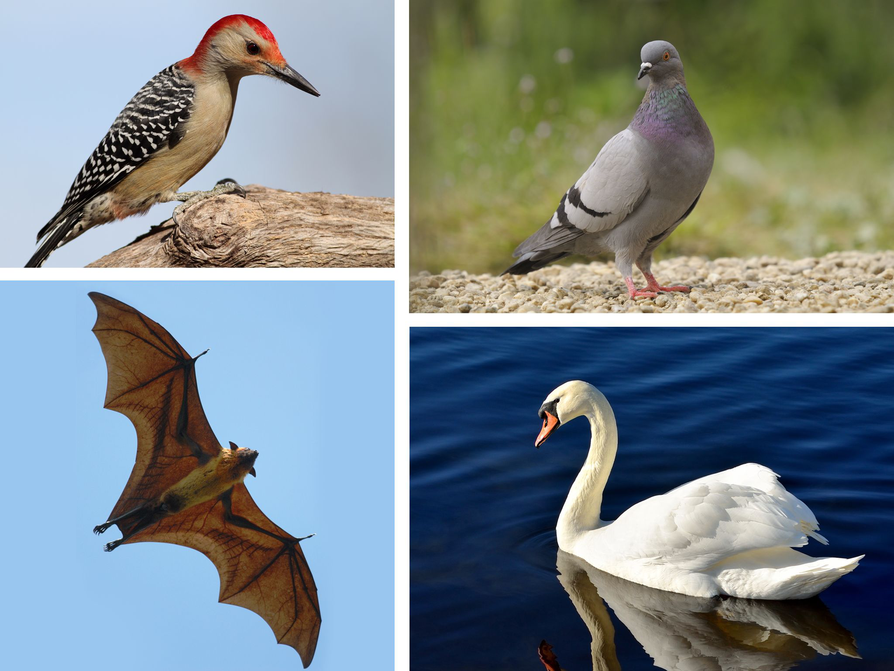# Lesson Guide

Have students individually predict whether the Strouhal number for a dolphin will fall within the range for birds and bats.

# Mathematical Practices

Mathematical Practice 3: Construct viable arguments and critique the reasoning of others.

Students construct a viable argument for the reasonableness of their solution and they have an opportunity to critique the work of others during the class discussion.

Mathematical Practice 4: Model with key concepts.

Students model the mathematics presented in the problem graphically.

Mathematical Practice 5: Use appropriate tools strategically.

Students use appropriate tools and problem-solving strategies to find the solution. Students may use a calculator as a possible tool for finding their solution.

Mathematical Practice 6: Attend to precision.

Students attend to precision while creating their graphs as well as when calculating the range in Strouhal numbers. Students also use precision when calculating the Strouhal number of a dolphin.

Mathematical Practice 7: Look for and make use of structure.

Students make use of the problem-solving structure while solving this problem.

# Interventions

Student has difficulty getting started.

• What information is provided in the problem? What are you trying to find?
• How can you organize the information presented in the problem?
• What problem-solving strategies can you use to help you find a solution?

Student has a solution, but it is incorrect.

• Does the information in your graph match the data in the table?
• How do you calculate the range for the data?

Student has a solution but is having difficulty articulating his or her thinking.

• How can you state the answer as a complete sentence?
• How would you describe your strategy to somebody who is struggling?

Student has a correct solution and is waiting on others to finish.

• If you were designing a flying robot with a high wing-flapping frequency, how would you design the length of the wings?
• Use the information given to create another problem for your partner to solve.

• Predictions will vary.
• $\frac{1.5\text{\hspace{0.17em}}·\text{\hspace{0.17em}}3}{15}=0.3$
The Strouhal number for the dolphin is 0.3. Comparisons to predictions will vary.

# A Dolphin

The dolphin has a cruising speed of 15 meters per second, an amplitude of 3 meters, and a flapping frequency of 1.5 beats per second.

• Predict whether the dolphin’s Strouhal number will fall within the range you calculated for the birds and bats.
• Calculate the Strouhal number for the dolphin and compare it to your prediction.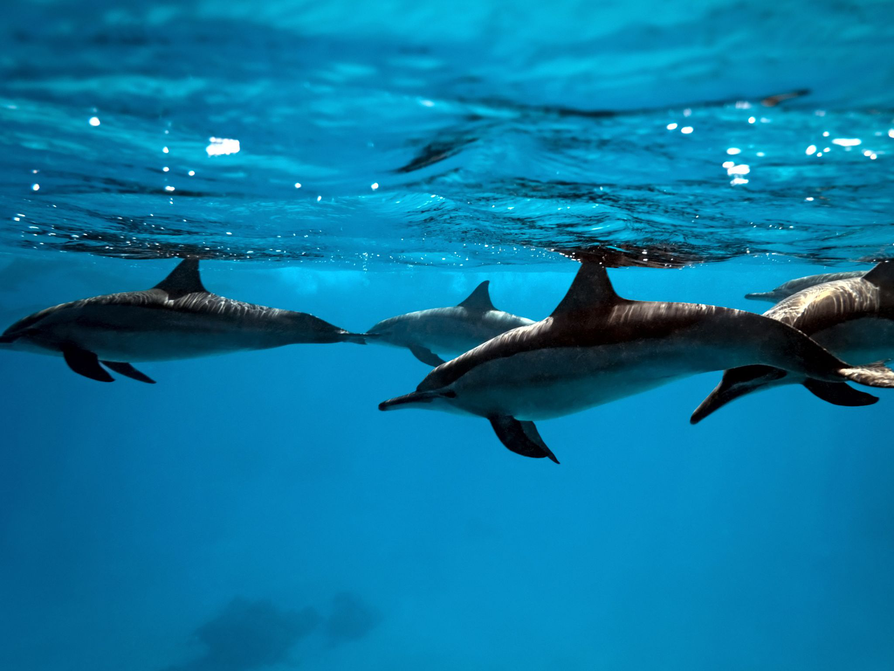# Preparing for Ways of Thinking

As pairs work, identify students who:

• Organize the information and describe the relationship among the variables in different ways.
• Generate different types of graphs. For example, horizontal bar graph, vertical bar graph, or line graph.
• Have different predictions about the Strouhal number for dolphins. For example, students look for students who thought that flying and swimming were similar and so the Strouhal numbers for dolphins would be similar as well, or students who thought there would be vast differences between traveling through the air and the water.
• Complete the Challenge Problem.

# Challenge Problem

• Answers will vary.Possible answer: If you were creating a flying robot, you would want the Strouhal number to fall within the range of other flying animals. Therefore, if the robot had a large amplitude, you would make the frequency shorter to fall within the correct range.

# Prepare a Presentation

Prepare a presentation of your findings. Justify all your findings with mathematical explanations.

# Challenge Problem

• Consider how the Strouhal numbers for flying and swimming animals are related.
• If you were designing a flying robot, how could this relationship help you with your design? Consider wing amplitude, frequency, and flying speed.

# Mathematics

Highlight the different strategies students used to solve the problems. Focus on how students created and implemented a problem-solving plan. Encourage discussion about the approaches for solving problems and the validity of the answers. Prompt students to give the presenters positive feedback as well as opportunities for improvement. Students should be refining their own strategies, correcting solutions, and taking notes during the presentations.

Point out that the range of the Strouhal numbers for flying and swimming animals is between 0.2 and 0.4. Flying and swimming are most efficient when the Strouhal number is between 0.2 and 0.4. Ask students how this range affects the variables in the problem, for example, if the flapping frequency increases, what happens to the amplitude? How does this affect the flying or swimming efficiency of the animal?

• How did you approach the problem? What strategies did you use?
• Do you think all Strouhal numbers fall within this range for flying and swimming animals? Why or why not?
• Do you think all swimming animals have the same relationship between flapping frequency and amplitude as the flying animals? Why?
• How did you create your graph? Are there other types of graphs that could display this information?
• Would a vertical bar graph show the information in the same way as a horizontal bar graph?
• How did you persevere in finding the solution?
• What was the most challenging aspect of the problem? How did you overcome this challenge?
• Are there multiple solutions to the problem? Why or why not?

Point out any mathematical processes you observed as students worked.

Have students who completed the Challenge Problem share their responses.

SWD: During Ways of Thinking, facilitate the learning process by encouraging students to discuss multiple strategies and representations of the mathematics. Ask questions and guide discussions. Help students to compare and contrast an animal's flying/swimming efficiency and their Strouhal number.

# Ways of Thinking: Make Connections

Take notes about other classmates’ graphs, and their use of the problem-solving process.